Updating search results...

# 28 Results

View
Selected filters:
• mass-cc-mathematics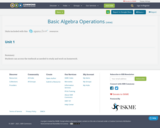Conditional Remix & Share Permitted
CC BY-NC
Rating
0.0 stars

Students can access the textbook as needed to study and work on homework.

Subject:
Algebra
Mathematics
Material Type:
Textbook
09/25/2019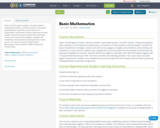Unrestricted Use
CC BY
Rating
0.0 stars

Topics include signed numbers, decimal numbers, exponential notation, scientific notation, solving and graphing linear equations, an introduction to polynomials, and systems of linear equations and their graphs. Geometrical topics include lines and angles, closed curves and convex polygons, triangles and similarities, and symmetry and proportion in nature and art. All course content by Valerie Dietel-Brenneman. Content added to OER Commons by Victoria Vidal.

Subject:
Mathematics
Numbers and Operations
Material Type:
Syllabus
Provider:
Roxbury Community College
05/10/2022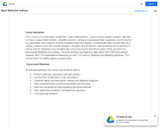Unrestricted Use
CC BY
Rating
0.0 stars

This course is a continuation of MAT087, Basic Mathematics. Topics include signed numbers, decimal numbers, exponential notation, scientific notation, solving and graphing linear equations, an introduction to polynomials, and systems of linear equations and their graphs. Geometrical topics include lines and angles, closed curves and convex polygons, triangles and similarities, and symmetry and proportion in nature and art. Students may complete this course during the first three weeks of the semester by passing the MyMathLab modules. Students will then be eligible to take either MAT 099 Intermediate Algebra, MAT 114-Quantitative Reasoning or MAT 120-Intro to Statistics the following semester. This course does not satisfy degree requirements.

Subject:
Mathematics
Material Type:
Full Course
Provider:
Northern Essex Community College
Author:
Jim Sullivan
05/15/2019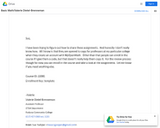Unrestricted Use
CC BY
Rating
0.0 stars

Topics include signed numbers, decimal numbers, exponential notation, scientific notation, solving and graphing linear equations, an introduction to polynomials, and systems of linear equations and their graphs. Geometrical topics include lines and angles, closed curves and convex polygons, triangles and similarities, and symmetry and proportion in nature and art. Students may complete this course during the first three weeks of the semester by passing the MyMathLab modules. Students will then be eligible to take either MAT 099 Intermediate Algebra, MAT 114-Quantitative Reasoning or MAT 120-Intro to Statistics the following semester. This course does not satisfy degree requirements. Students may complete this course during the first three weeks of the semester by passing the MyOpenMath Acceleration assignments.

Subject:
Mathematics
Material Type:
Full Course
Provider:
Roxbury Community College
Author:
Valerie Dietel-Brenneman
05/15/2019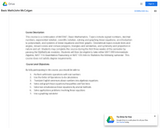Unrestricted Use
CC BY
Rating
0.0 stars

This course is a continuation of MAT087, Basic Mathematics. Topics include signed numbers, decimal numbers, exponential notation, scientific notation, solving and graphing linear equations, an introduction to polynomials, and systems of linear equations and their graphs. Geometrical topics include lines and angles, closed curves and convex polygons, triangles and similarities, and symmetry and proportion in nature and art. Students may complete this course during the first three weeks of the semester by passing the MyMathLab modules. Students will then be eligible to take either MAT 099 Intermediate Algebra, MAT 114-Quantitative Reasoning or MAT 120-Intro to Statistics the following semester. This course does not satisfy degree requirements.

Subject:
Mathematics
Material Type:
Full Course
Provider:
Roxbury Community College
Author:
John McColgan
05/15/2019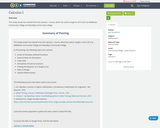Unrestricted Use
CC BY
Rating
0.0 stars

This Study Guide has evolved from the Calculus 1 course, which the author taught in 2015-2019 at Middlesex Community College and MassBay Community College.

Subject:
Mathematics
Material Type:
Lecture Notes
Author:
Igor Baryakhtar
06/14/2020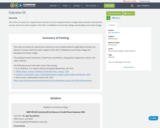Unrestricted Use
CC BY
Rating
0.0 stars

This is the curriculum for a asynhronous Calculus III course implemented for an eight-week semester and based on courses, which the author taught in 2016-2021 at Middlesex Community College and MassBay Community College.&nbsp;

Subject:
Mathematics
Material Type:
Full Course
Author:
Igor Baryakhtar
06/30/2021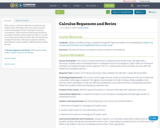Unrestricted Use
CC BY
Rating
0.0 stars

This course is a brief introduction to sequences and infinite series. We begin with a discussion of power series and develop tests for convergence and non-convergence. Taylor series are introduced and lead to an analysis of power series in general. This is a 1-credit course that can be taken any time after the student has completed Calculus I. All course content created by Javad Moulai. Content added to OER Commons by Julia Greider.

Subject:
Calculus
Mathematics
Material Type:
Homework/Assignment
Textbook
Provider:
Roxbury Community College
04/26/2022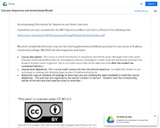Unrestricted Use
CC BY
Rating
0.0 stars

This course is a brief introduction to sequences and infinite series. We begin with a discussion of power series and develop tests for convergence and non-convergence. Taylor series are introduced and lead to an analysis of power series in general. This is a 1-credit course that can be taken any time after the student has completed Calculus I.

Subject:
Calculus
Mathematics
Material Type:
Full Course
Provider:
Roxbury Community College
Author:
05/15/2019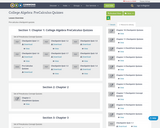Unrestricted Use
CC BY
Rating
0.0 stars

Pre-calculus problem sets and quizzes.CC x BY Lauren Brewer

Subject:
Algebra
Material Type:
Assessment
Author:
Lauren Brewer
01/02/2020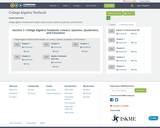Unrestricted Use
CC BY
Rating
0.0 stars

College Algebra Textbook with chapters about&nbsp;Linears, Systems, Quadratics, and FunctionsCC x BY Ben Atchison

Subject:
Algebra
Material Type:
Lecture Notes
Textbook
Author:
Ben Atchison
01/02/2020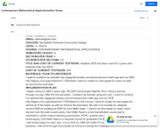Unrestricted Use
CC BY
Rating
0.0 stars

Full course on Contemporary Mathematical Applications.

Subject:
Mathematics
Material Type:
Full Course
Provider:
Springfield Technical Community College
Author:
Ann Simao
05/15/2019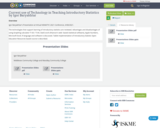Unrestricted Use
CC BY
Rating
0.0 stars

Igor Baryakhtar&#39;s Presentation at Virtual NEMATYC 2021 Conference, 4/08/2021.The technologies that support learning of introductory statistics are reviewed. Advantages and disadvantages of using Graphing calculator TI 83 / TI 84, StatCrunch (Pearson&#39;s web- based statistical software), Apple Numbers, Microsoft Excel, R language and software is discussed. Tablet implementation of Introductory Statistic Open Education Resources based course is described.

Subject:
Mathematics
Material Type:
Teaching/Learning Strategy
Author:
Igor Baryakhtar
04/11/2021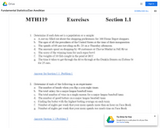Unrestricted Use
CC BY
Rating
0.0 stars

Introductory Statistics Course covering hypothesis testing, confidence interval, sampling, probability, counting techniques, correlation, linear regression, data collection and more.

Subject:
Mathematics
Statistics and Probability
Material Type:
Full Course
Provider:
Bristol Community College
Author:
Dan Avedikian
05/01/2019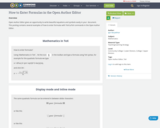Unrestricted Use
CC BY
Rating
0.0 stars

Open Author Editor gives an opportunity to write beautiful equations and symbols easily in your&nbsp; document.This posting contains several examples of how to enter formulas with TeX/LaTeX commands in the Open Author Editor.&nbsp;

Subject:
Mathematics
Material Type:
Teaching/Learning Strategy
Author:
Igor Baryakhtar
03/07/2021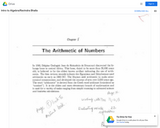Unrestricted Use
CC BY
Rating
0.0 stars

Introductory Algebra is the second of three classes in the developmental mathematics sequence. It provides a development of concepts of variables, expressions, and equations using symbolic algebra to represent primarily linear relationships both graphically and analytically. The concept of function will be developed for the application of linear equations and concepts of dependent and independent variable. Students will also learn to solve simultaneous linear equations as well as how to construct linear equations from slope and point information. Application problems will include geometric figure quantities, ratio and proportion, direct and indirect variation, and conversion of units. Finding the greatest common factor of a polynomial will also be included. The emphasis of the three semester sequence is fortification of mental calculation power with minimum reliance on digital calculation.

Subject:
Algebra
Mathematics
Material Type:
Full Course
Provider:
MassBay Community College
Author:
Ravindra Bhalla
05/13/2019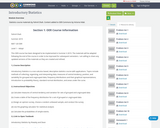Unrestricted Use
CC BY
Rating
0.0 stars

&nbsp; Introductory Statistics is a non-calculus based, descriptive statistics course with applications. Topics include methods of collecting, organizing, and interpreting data; measures of central tendency, position, and variability for grouped and ungrouped data; frequency distributions and their graphical representations; introduction to probability theory, standard normal distribution, and areas under the curve. Course materials created by Fahmil Shah, content added to OER Commons by Victoria Vidal.

Subject:
Mathematics
Statistics and Probability
Material Type:
Module
Author:
Fahmil Shah
11/06/2019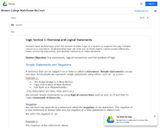Unrestricted Use
CC BY
Rating
0.0 stars

Survey course for college mathematics.

Subject:
Mathematics
Material Type:
Full Course
Provider:
Bristol Community College
Author:
Susan McCourt
05/01/2019Unrestricted Use
Public Domain
Rating
0.0 stars

The New England Mathematical Association of Two Year Colleges 2021 Virtual Conference.
(Monday, April 5 - Friday, April 16)
Recorded presentations

Subject:
Mathematics
Material Type:
Primary Source
04/23/2021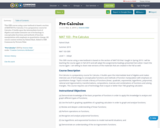Unrestricted Use
CC BY
Rating
0.0 stars

This OER course using a new textbook is based a section of MAT103 Pre-Calculus. It is a preparatory course for Calculus. It builds upon the intermediate level of Algebra and makes intensive use of technology to conceptualize functions and methods of function manipulation with emphasis on quantitative change. All course content written by Fahmil Shah. Added to OER Commons by Victoria Vidal.

Subject:
Algebra
Calculus
Functions
Mathematics
Trigonometry
Material Type:
Full Course
Provider:
Roxbury Community College